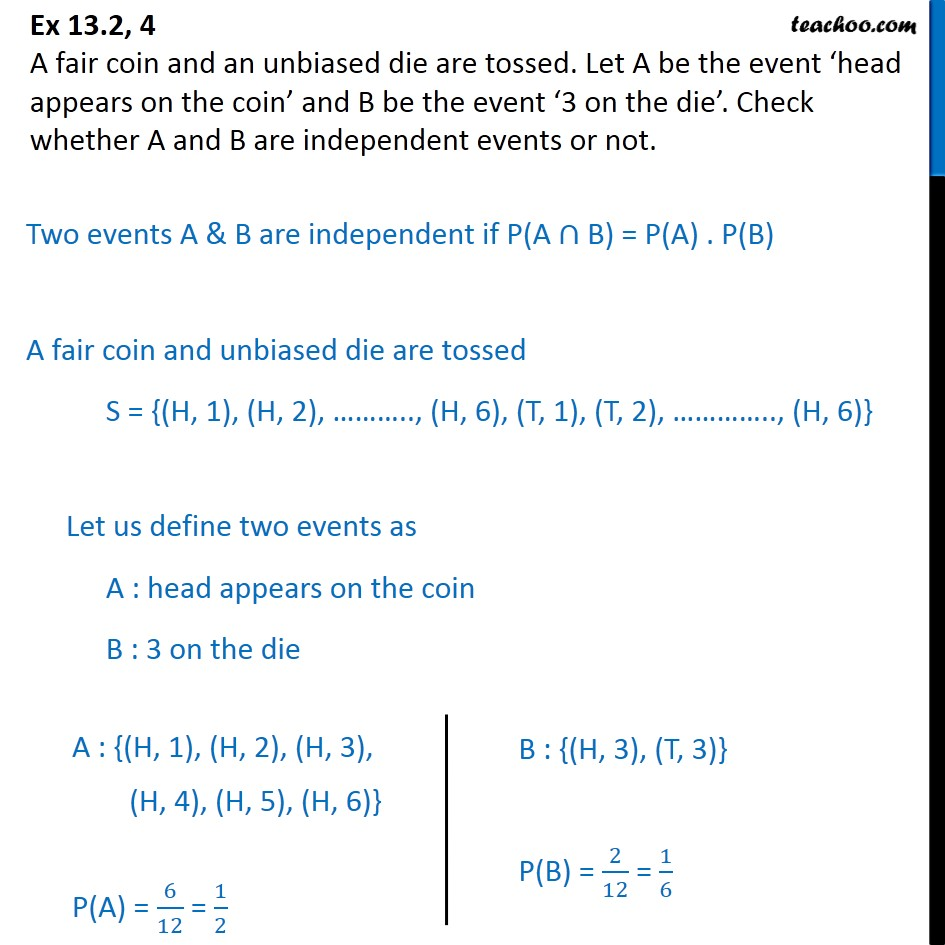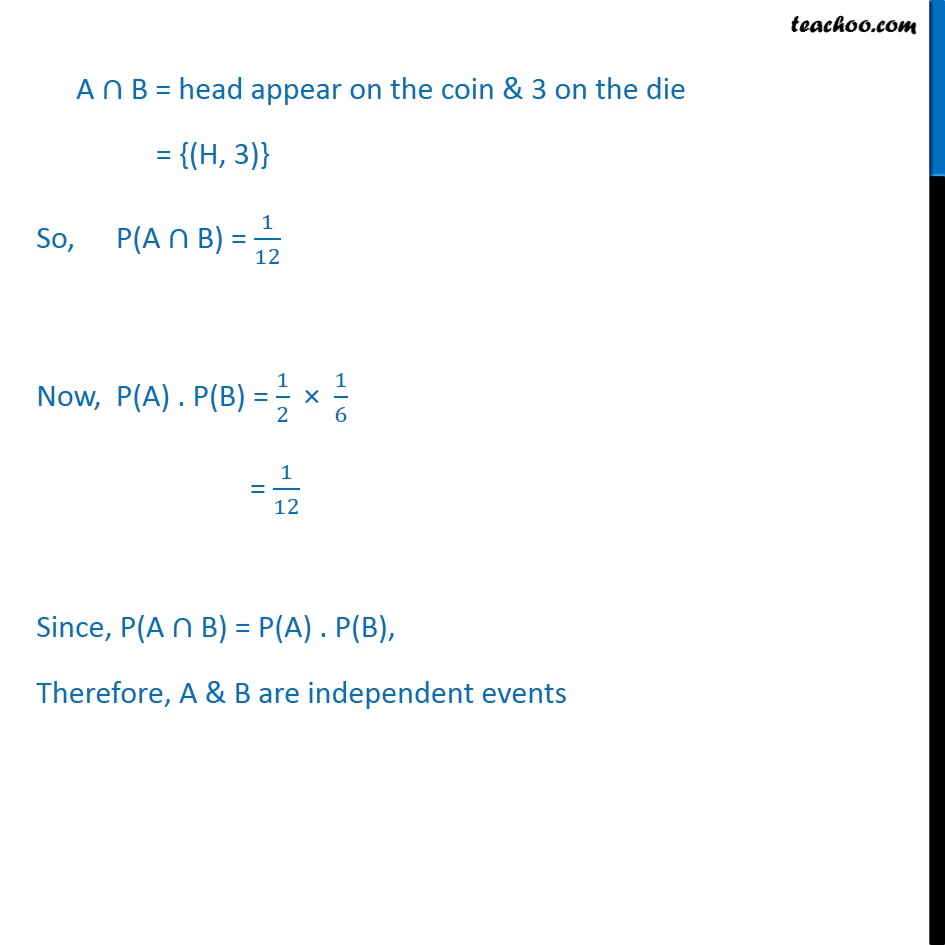Ex 13.2

Chapter 13 Class 12 Probability
Serial order wiseGet live Maths 1-on-1 Classs - Class 6 to 12

### Transcript

Ex 13.2, 4 A fair coin and an unbiased die are tossed. Let A be the event ‘head appears on the coin’ and B be the event ‘3 on the die’. Check whether A and B are independent events or not. Two events A & B are independent if P(A ∩ B) = P(A) . P(B) A fair coin and unbiased die are tossed S = {(H, 1), (H, 2), ……….., (H, 6), (T, 1), (T, 2), ………….., (H, 6)} Let us define two events as A : head appears on the coin B : 3 on the die A ∩ B = head appear on the coin & 3 on the die = {(H, 3)} So, P(A ∩ B) = 1﷮12﷯ Now, P(A) . P(B) = 1﷮2﷯ × 1﷮6﷯ = 1﷮12﷯ Since, P(A ∩ B) = P(A) . P(B), Therefore, A & B are independent events